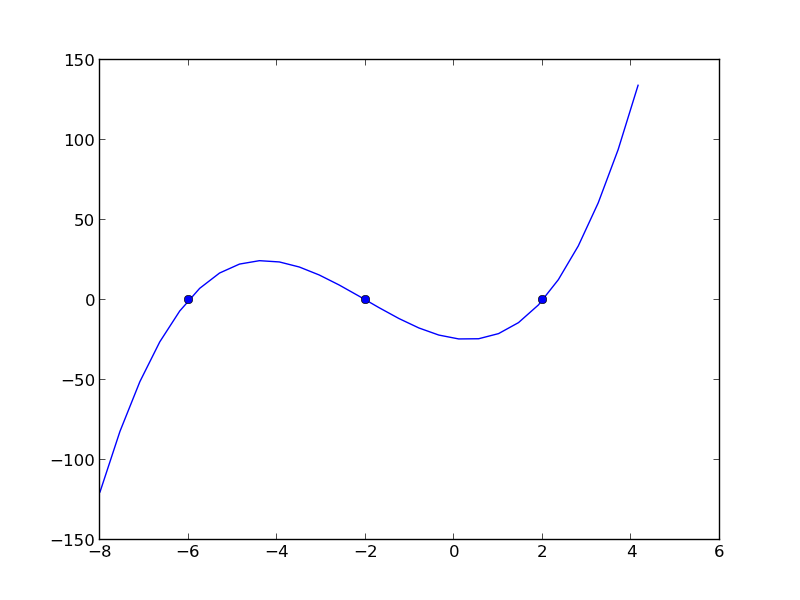## Mimicking ode events in python

| categories: ode | tags: | View Comments

The ODE functions in scipy.integrate do not directly support events like the functions in Matlab do. We can achieve something like it though, by digging into the guts of the solver, and writing a little code. In previous example I used an event to count the number of roots in a function by integrating the derivative of the function.

import numpy as np
from scipy.integrate import odeint

def myode(f, x):
return 3*x**2 + 12*x -4

def event(f, x):
'an event is when f = 0'
return f

# initial conditions
x0 = -8
f0 = -120

# final x-range and step to integrate over.
xf = 4   #final x value
deltax = 0.45 #xstep

# lists to store the results in
X = [x0]
sol = [f0]
e = [event(f0, x0)]
events = []
x2 = x0
# manually integrate at each time step, and check for event sign changes at each step
while x2 <= xf: #stop integrating when we get to xf
x1 = X[-1]
x2 = x1 + deltax
f1 = sol[-1]

f2 = odeint(myode, f1, [x1, x2]) # integrate from x1,f1 to x2,f2
X += [x2]
sol += [f2[-1]]

# now evaluate the event at the last position
e += [event(sol[-1], X[-1])]

if e[-1] * e[-2] < 0:
# Event detected where the sign of the event has changed. The
# event is between xPt = X[-2] and xLt = X[-1]. run a modified bisect
# function to narrow down to find where event = 0
xLt = X[-1]
fLt = sol[-1]
eLt = e[-1]

xPt = X[-2]
fPt = sol[-2]
ePt = e[-2]

j = 0
while j < 100:
if np.abs(xLt - xPt) < 1e-6:
# we know the interval to a prescribed precision now.
# print 'Event found between {0} and {1}'.format(x1t, x2t)
print 'x = {0}, event = {1}, f = {2}'.format(xLt, eLt, fLt)
events += [(xLt, fLt)]

m = (ePt - eLt)/(xPt - xLt) #slope of line connecting points
#bracketing zero

#estimated x where the zero is
new_x = -ePt / m + xPt

# now get the new value of the integrated solution at that new x
f  = odeint(myode, fPt, [xPt, new_x])
new_f = f[-1][-1]
new_e = event(new_f, new_x)

# now check event sign change
if eLt * new_e > 0:
xPt = new_x
fPt = new_f
ePt = new_e
else:
xLt = new_x
fLt = new_f
eLt = new_e

j += 1

import matplotlib.pyplot as plt
plt.plot(X, sol)

# add event points to the graph
for x,e in events:
plt.plot(x,e,'bo ')
plt.savefig('images/event-ode-1.png')

x = -6.00000006443, event = -4.63518112781e-15, f = -4.63518112781e-15
x = -1.99999996234, event = -1.40512601554e-15, f = -1.40512601554e-15
x = 1.99999988695, event = -1.11022302463e-15, f = -1.11022302463e-15That was a lot of programming to do something like find the roots of the function! Below is an example of using a function coded into pycse to solve the same problem. It is a bit more sophisticated because you can define whether an event is terminal, and the direction of the approach to zero for each event.

from pycse import *
import numpy as np

def myode(f, x):
return 3*x**2 + 12*x -4

def event1(f, x):
'an event is when f = 0 and event is decreasing'
isterminal = True
direction = -1
return f, isterminal, direction

def event2(f, x):
'an event is when f = 0 and increasing'
isterminal = False
direction = 1
return f, isterminal, direction

f0 = -120

xspan = np.linspace(-8, 4)
X, F, TE, YE, IE = odelay(myode, f0, xspan, events=[event1, event2])

import matplotlib.pyplot as plt
plt.plot(X, F, '.-')

# plot the event locations.use a different color for each event
colors = 'rg'

for x,y,i in zip(TE, YE, IE):
plt.plot([x], [y], 'o', color=colors[i])

plt.savefig('images/event-ode-2.png')
plt.show()
print TE, YE, IE

[-6.0000001083101306, -1.9999999635550625] [-3.0871138978483259e-14, -7.7715611723760958e-16] [1, 0]Copyright (C) 2013 by John Kitchin. See the License for information about copying.

org-mode source